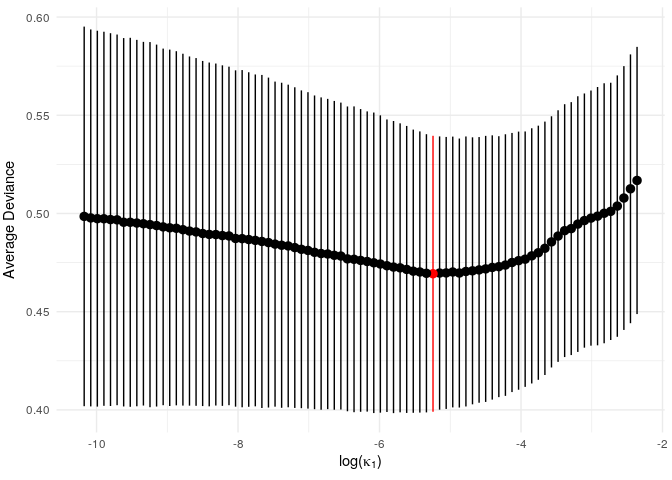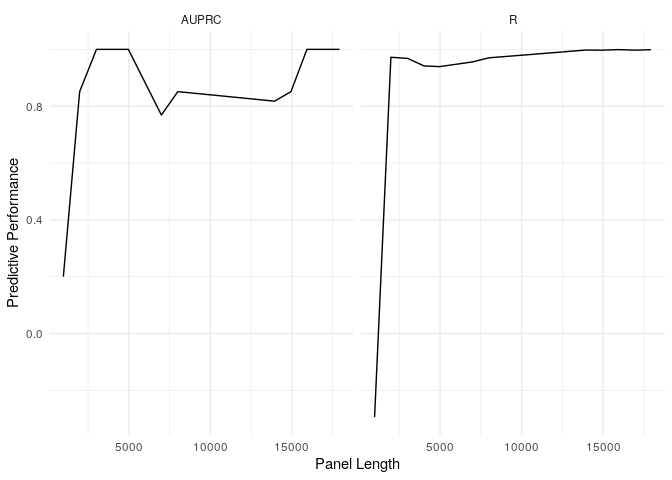# ICBioMark

Welcome to ICBioMark, based on the paper “Data-driven design of targeted gene panels for estimating immunotherapy biomarkers”, Bradley and Cannings (see our preprint).

## Installation

You can install the release version of ICBioMark from CRAN with:

``install.packages("ICBioMark")``

Alternatively, you can install the development version of this package from this github repository (using the devtools package) with:

``devtools::install_github("cobrbra/ICBioMark")``

## Examples

Upon installation we can load the package.

``library(ICBioMark)``

To demonstrate the typical workflow for using ICBioMark, we play around with a small and friendly example dataset. This is pre-loaded with the package, but just comes from the data simulation function `generate_maf_data()`, so you can play around with datasets of different sizes and shapes.

### Input Data

Our example dataset, called `example_maf_data`, is a list with two elements: `maf` and `gene_lengths`. These are the two pieces of data that you’ll always need to use this package, and they look as follows:

• `maf` is a data frame in the MAF (mutation annotated format) style. For a set of sequenced tumour/normal pairs, this means a table with a row for every mutation identified, with columns corresponding to properties such as the sample ID for the tumour of origin, the gene, chromosome and nucelotide location of the mutation, and the type of mutation observed. In the real world, MAF datasets often have lots of extra information beyond this, but in our small example we’ve just included sample, gene and mutation type (it’s all we’ll need!). The top five rows look like this:
`````` # example_maf_data <- generate_maf_data()

Tumor_Sample_Barcode Hugo_Symbol Variant_Classification
SAMPLE_96 GENE_14 Missense_Mutation
SAMPLE_73 GENE_14 Frame_Shift_Ins
SAMPLE_55 GENE_4 Missense_Mutation
SAMPLE_96 GENE_3 Missense_Mutation
SAMPLE_38 GENE_7 Missense_Mutation
• `gene_lengths`, another data frame, this time containing the names of genes that you’ll want to include in your modelling and their length. Gene length is a complex and subtle thing to define - we advise using coding length as defined in the Ensembl database. For this example, however, gene lengths are again randomly chosen:
``  kable(head(example_maf_data\$gene_lengths, 5), row.names = FALSE)``
Hugo_Symbol max_cds
GENE_1 961
GENE_2 1009
GENE_3 1011
GENE_4 976
GENE_5 1016

These are the only two bits of data required to use ICBioMark. Your gene lengths data can contain values for far more genes than are observed in your dataset, and it’s not a huge problem if a couple of genes in a Whole-Exome Sequencing (WES) experiment are missing gene length information, but lots of missing values will cause issues with your model accuracy. Later versions of this package should be able to address missing gene length data.

### Train/Val/Test and Matrix Construction

The MAF format is widely used and standardised, but not especially helpful for our purposes. The ideal format for our data is a matrix, where every row corresponds to a sample, every column corresponds to a gene/mutation type combination, and each entry corresponds to how many mutations of that sample, gene and type were sequenced. At the same time as this, we’d like to separate our training data from separately reserved validation and test data. We do this using the function `get_mutation_tables()`.

Before we do this, however, we need to talk about mutation types. Our procedure models different mutation types separately, so in theory one could have separate parameters for each mutation type (e.g. ‘Missense_Mutation’ or ‘Frame_Shift_Ins’). However, doing so will vastly increase the computational complexity of fitting a generative model. It is also not particularly informative to fit parameters to extremely scarce mutation types. We therefore group mutation types together (and can filter some out if we don’t want to include them in our modelling). This will happen behind-the-scenes, but is worth knowing about to understand the outputs generated. Mutations types are grouped and filtered by the function `get_mutation_dictionary()`. In general we recommend separately modelling indel mutations (so that we can predict TIB later), synonymous mutations (as these don’t count towards TMB or TIB), and lumping together all other nonsynonymous mutation types. The function `get_mutation_dictionary()` produces a list of mutation types, with labels for their groupings. For example:

``  kable(get_mutation_dictionary(), col.names = "Label")``
Label
Missense_Mutation NS
Nonsense_Mutation NS
Splice_Site NS
Translation_Start_Site NS
Nonstop_Mutation NS
In_Frame_Ins NS
In_Frame_Del NS
Frame_Shift_Del I
Frame_Shift_Ins I
Silent S
Splice_Region S
3’Flank S
5’Flank S
Intron S
RNA S
3’UTR S
5’UTR S

We’ve given each mutation type one of three labels: “NS”, “S” and “I”. We could have excluded synonymous mutation types by using `get_mutation_dictionary(include_synonymous = FALSE)`.

Now we can produce our training, validation and test sets (again for this example workflow these are pre-loaded). The object produced has three elements: ‘train’, ‘val’ and ‘test’. Each of these contains a sparse mutation matrix (‘matrix’) and other information describing the contents of the matrix (‘sample_list’, ‘gene_list’, ‘mut_types_list’ and ‘col_names’). We can see that the list of mutation types contains the three labels we specified above

``````  #example_tables <- get_mutation_tables(example_maf_data\$maf,
#                   sample_list = paste0("SAMPLE_", 1:100))

print(example_tables\$train\$mut_types_list)
#>  "NS" "I"  "S"``````

and that the columns of each matrix correspond to each combination of mutation type and gene:

``````  print(head(example_tables\$train\$col_names, 10))
#>   "GENE_1_NS" "GENE_1_I"  "GENE_1_S"  "GENE_2_NS" "GENE_2_I"  "GENE_2_S"
#>   "GENE_3_NS" "GENE_3_I"  "GENE_3_S"  "GENE_4_NS"``````

### Fitting the Generative Model

There are relatively few decisions left to be made at this point: all we need to do to fit a generative model is to provide gene lengths data and training data to the function `fit_gen_model()`. We can visualise output of our model with `vis_model_fit()`.

``````  # example_gen_model <- fit_gen_model(example_maf_data\$gene_lengths,
#                       table = example_tables\$train)

print(vis_model_fit(example_gen_model))``````Since this is a small example, we don’t get a particularly strong signal, but we do see an optimum level of penalisation. (NB: the function `vis_model_fit()` essentially performs the same task as `glmnet::plot.cv.glmnet()`. In later versions the `glmnet` version will hopefully be directly applicable and `vis_model_fit()` will be redundant).

### Fitting the Predictive Model

We now construct a first-fit predictive model. The parameter `lambda` controls the sparsity of each iteration, so it may take some experimentation to get a good range of panel lengths.

``````  example_first_pred_tmb <- pred_first_fit(example_gen_model,
lambda = exp(seq(-9, -14, length.out = 100)),
training_matrix = example_tables\$train\$matrix,
gene_lengths = example_maf_data\$gene_lengths)``````

From this we can construct a range of refit estimators:

``````  example_refit_range <- pred_refit_range(pred_first = example_first_pred_tmb,
gene_lengths = example_maf_data\$gene_lengths)``````

### Making Predictions and Analysing Performance

With a predictive model fitted, we can use the function `get_predictions()` along with a new (validation or test) dataset to produce predictions on that dataset. We then provide several functions including `get_stats()` to analyse the output compared to true values.

``````  example_refit_range %>%
get_predictions(new_data = example_tables\$val) %>%
get_stats(biomarker_values = example_tmb_tables\$val, model = "T", threshold = 10) %>%
ggplot(aes(x = panel_length, y = stat)) + geom_line() + facet_wrap(~metric) + theme_minimal() + labs(x = "Panel Length", y = "Predictive Performance")``````## Getting Help

Please do feel free to flag issues and requests on this repository. You can also email me.